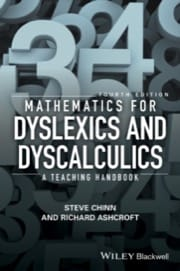# Mathematics for Dyslexics and Dyscalculics, 4ed: A Teaching Handbook

\$71.95  Paperback360 pages
2017
ISBN: 9781119159964

A seminal handbook in the field for more than 20 years, this new and updated edition of Mathematics for Dyslexics and Dyscalculics contains the latest research and best practices for helping learners with numerical and mathematical difficulties.

• Provides a complete overview of theory and research in the fields of dyslexia and dyscalculia, along with detailed yet pragmatic methods to apply in the classroom
• Contains enhanced coverage of place value and the role of the decimal point, why fractions can challenge a developed logic for arithmetic, and the complexity of time along with new material on addressing anxiety, fear, motivation, and resilience in the classroom; and links to new resources including standardised tests and recommended reading lists
• Written by two mathematics teachers with 50 years of teaching experience between them, much of it in specialist settings for students with specific learning difficulties
• Offers effective teaching strategies for learners of all ages in a structured but accessible format

Chapter 1: Dyscalculia, Dyslexia and Mathematics.

• Introduction.
• Definitions of Dyslexia.
• Dyscalculia.
• The Nature of Mathematics.

Chapter 2: Factors that may Contribute to Learning Difficulties in Mathematics.

• Potential Areas of Difficulty in Learning Mathematics.
• General Principles of Intervention.
• Summary.

Chapter 3: Cognitive (Thinking) Style in Mathematics Introduction.

• Qualitative and Quantitative Style.
• Cognitive (Thinking) Style in the Classroom Grasshoppers (Intuitive/Answer Oriented) and Inchworms (Step by Step/Formula Oriented).
• Examples.
• Summary.

Chapter 4: Testing and Diagnosis.

• A Diagnostic Test Protocol.
• Structure of the Diagnostic Protocol.
• Attitude and Anxiety.
• Thinking Style or Cognitive Style.
• Summary of the Test Protocol.
• Testing for Dyscalculia.

Chapter 5: Concept of Number.

• Introduction.
• Early Recognition of Numbers and Their Values.
• The Language of Mathematics.
• Early Number Work.
• Visual Sense of Number.
• Visual Clues to the Number Concept.
• Number Bonds.
• Place Value.
• Number Bonds for 10.
• Numbers near 10, 100 or 1000.
• Summary.

Chapter 6: Addition and Subtraction: Basic Facts.

• Introduction.
• Strategies for Learning/Remembering the Addition and Subtraction Facts.
• Summary.
• Subtraction Facts.
• Extension.

Chapter 7: Times Tables.

• Introduction.
• Rote Learning with Audio Recorders and Computers.
• Learning by Understanding.
• The Commutative Property.
• Learning the Table Square.
• Summary.

Chapter 8: Computational Procedures for Addition and Subtraction.

• Estimation.
• Teaching Subtraction as a Separate Exercise.

Chapter 9: Multiplication.

• Introduction.
• The Special Case of Multiplying by 10 and Powers of 10.
• Multiplication.
• Estimation.
• Extension.

Chapter 10: Division.

• Introduction.
• Introduction to Division.
• Estimating.
• Division by Powers of 10.
• Division by Multiples of Powers of ten.
• Conclusion.

Chapter 11: Fractions, Decimals and Percentages: An Introduction.

• Introduction.
• Fractions.
• Decimals.
• Percentages.
• A Global Model for Percentages, Fractions and Decimals.
• A Global Exercise with Fractions, Percentages and Decimals.

Chapter 12: Operating with Fractions.

• Introduction.
• Making Segment Sizes the Same.
• Comparing Fractions.
• Combining Fractions.
• Subtracting Fractions.
• Multiplying by Fractions.
• Multiplying Mixed Fractions.
• Dividing with Fractions.

Chapter 13: Decimals.

• Introduction.
• Multiplication and Division by Powers of 10.
• Division of Decimals.
• Summary.

Chapter 14: Percentages.

• Introduction.
• An Image of Percentage.
• Summary.

Chapter 15: Time.

• What are the Potential Problems with Time?
• Time Problems.
• Summary.

Chapter 16: Teaching the Full Curriculum.

• Introduction.
• Some General Principles.
• Teaching the Other Parts of the Curriculum.
• Combining the Parts of the Curriculum.
• Summary.

Chapter 17: Attacking and Checking Questions.

• Practice Examples.
• Preliminary Checks.
• Attacking Questions.
• Methods of attacking questions.
• Checking.

Chapter 18: Important Elements of a Teaching Programme.

• Introduction.
• Consider the Pupils’ Needs.
• The Structure of the Course.
• Classroom Management: Making the Lessons Suit the Pupils.
• Evolving Expectations and Emphases.
• Internal Assessment.
• GCSE Examinations.
• Summary.

Appendices.

• Appendix 1: Books, Journals, Tests and Games.
• Appendix 2: Teaching Materials.

References.

Index.